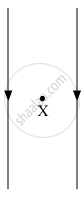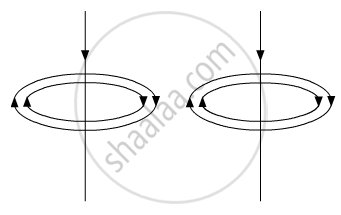# The following diagram shows two parallel straight conductors carrying same current. Copy the diagram and draw the pattern of the magnetic field lines around them showing their directions. - Science

Diagram

The following diagram shows two parallel straight conductors carrying the same current. Copy the diagram and draw the pattern of the magnetic field lines around them showing their directions. What is the magnitude of the magnetic field at a point 'X' which is equidistant from the conductors? Give justification for your answer.#### Solution

Applying Right-hand thumb rule, we can draw the magnetic field lines around the given wires. As both the wires have a current flowing downward hence the direction of the magnetic field will be clockwise for both of them as shown in the figure:At point X, the magnetic field due to both conductors have opposite direction and as the point is equidistant from the conductors so due to symmetry the magnetic field the net magnitude of the magnetic field at point X is zero.

Concept: Force on a Current Carrying Conductor in a Magnetic Field
Is there an error in this question or solution?